Could you further explain your explanation of Practice Question #4 in PWN the SAT Math Guide “Polynomials” chapter (p. 148). In your long division confirmation of the answer (explanation p. 326), how do you know to divide by 9x + 1?

Sure. Here’s the question:

First, as I say in the solution, all you really need to do here is pay attention to the leading terms. When you divideby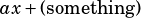and get, then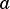must be 9.

When I check by doing long division, I usebecause I’m trying to confirm that the equation works if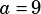. As shown in the solution, the long division of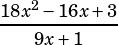works out to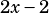with a remainder of 5—or. That matches the right hand side of the original equation.